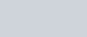Find a particular solution satisfying the given condition for each of the following differential equations.
Question:

Find a particular solution satisfying the given condition for each of the following differential equations.

$\frac{d y}{d x}+2 y=e^{-2 x} \sin x$, given that $y=0$, when $x=0$

Solution: